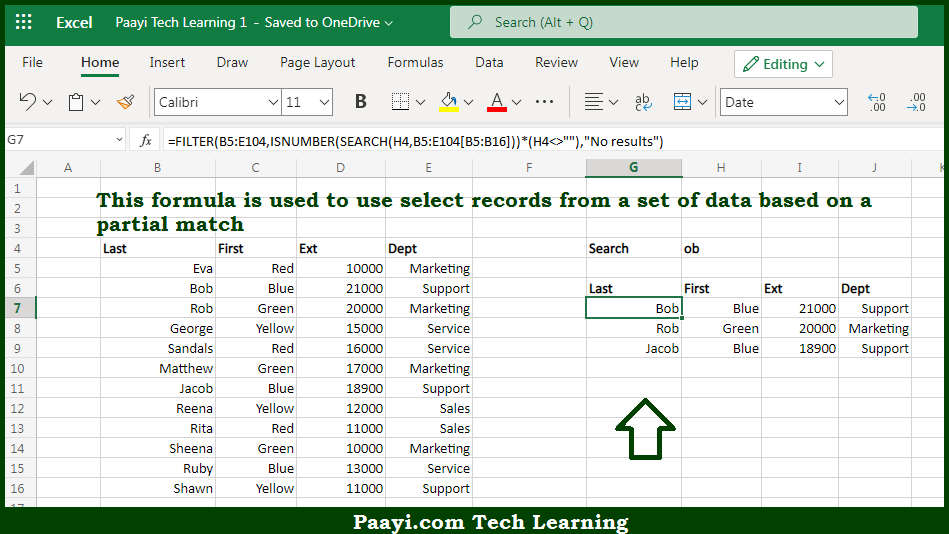# Learn How to Filter With Partial Match in Microsoft Excel

Written by | 0 Comments | 1679 Views

In this article, you will learn how to evaluate things in Dynamic Arrays with formulas in Microsoft Excel using a single/combination(s) of functions. You will also know How to Filter With Partial Match and see the generic formula.

Learn How to Filter With Partial Match in Microsoft Excel

The main purpose of this formula is to select records from a set of data based on a partial match. Here we will learn how to filter with a partial match in the given data range in the workbook in Microsoft Excel. That implies, with the help of a formula based on the FILTER, and SEARCH function you can able to use select records from a set of data based on a partial match. So, with the help of this formula, you can able to filter with a partial match in the given data range in the workbook in Microsoft Excel.

General Formula to Filter With Partial Match

The Explanation for the Filter With Partial MatchSo we know that with the help of the given formula above you can able to select records from a set of data based on a partial match. Here we will learn how to filter with the partial match in the given data range in the workbook in Microsoft Excel. As we know that the goal is to extract a set of records that match a partial text string. To put things simply, we are only matching one field in the data, the "last name." FILTER function is doing the core operation, which extracts matching data from a range based on a logical filter. So, with the help of this formula, you can able to select records from a set of data based on a partial match. Here we will learn how to filter with a partial match in the given data range in the workbook in Microsoft Excel.